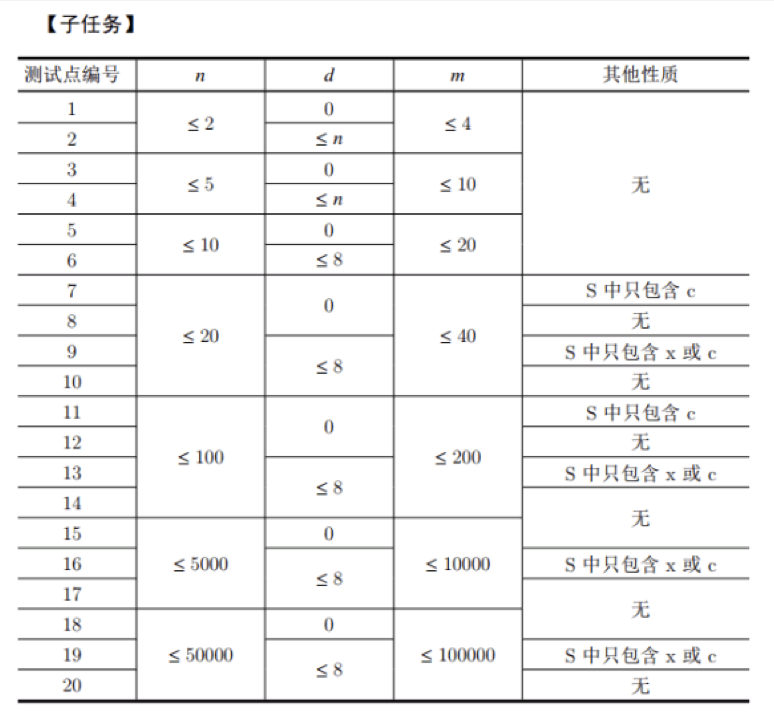【NOI2017】游戏 2-SATNekoMio 6月 29, 2018
• 在其它设备中阅读本文章
•题目描述

$n$ 场游戏的地图可以用一个小写字母组成的字符串描述。例如：$S=\texttt{xaabxcbc}$ 表示小L计划进行 $8$ 场游戏，其中第 $1$ 场和第 $5$ 场的地图类型是 $x$，适合所有赛车，第 $2$场和第 $3$ 场的地图是 $a$，不适合赛车 $A$，第 $4$ 场和第 $7$ 场的地图是 $b$，不适合赛车 $B$，第 $6$ 场和第 $8$ 场的地图是 $c$，不适合赛车 $C$。

输入格式

$n$, $d$, $S$ 的含义见题目描述，其中 $S$ 包含 $n$ 个字符，且其中恰好 $d$ 个为小写字母 $x$。

样例

样例输入

3 1
xcc
1
1 A 2 B


样例输出

ABA


数据范围与提示题解

2-SAT

$a, b, c$ 的时候是2-SAT， 那 $x$ 不就是 3-SAT 了么。

2-SAT 输出方案

Tarjan

Tarjan缩点时会给联通块编号

#pragma GCC optimize("O3")
#include <bits/stdc++.h>
using namespace std;
int x = 0, f = 1;
char ch = getchar();
while (ch < '0' || ch > '9') {
if (ch == '-') f = -1;
ch = getchar();
}
while (ch >= '0' && ch <= '9') {
x = x * 10 + ch - '0';
ch = getchar();
}
return x * f;
}
const int MAXN = 50005;
const int MAXM = 100005;
struct data {
int i, hi, j, hj;
} D[MAXM];
struct edge {
int END, next;
} v[MAXM << 2];
int first[MAXN << 1], p;
void add(int a, int b) {
v[p].END = b;
v[p].next = first[a];
first[a] = p++;
}
char tmp;
char S[MAXN];
int id;
int ID(int x, int y) {
if (S[x] - 'a' == y) return 0;
if (S[x] == 'a') return x * 2 + y - 1;
else if (S[x] == 'b') return x * 2 + y / 2;
else return x * 2 + y;
}
int dfn[MAXN << 1], low[MAXN << 1], Index, belong[MAXN << 1], T;
bool vis[MAXN << 1];
int st[MAXN << 1], top;
void Tarjan(int rt) {
dfn[rt] = low[rt] = ++Index;
st[++top] = rt;
vis[rt] = 1;
for (int i = first[rt]; i != -1; i = v[i].next) {
if (!dfn[v[i].END]) {
Tarjan(v[i].END);
low[rt] = min(low[rt], low[v[i].END]);
} else if (vis[v[i].END])
low[rt] = min(low[rt], dfn[v[i].END]);
}
if (low[rt] == dfn[rt]) {
T++;
int x;
do {
x = st[top--];
vis[x] = 0;
belong[x] = T;
} while (low[x] != dfn[x]);
}
}
bool Calc(int n, int m) {
memset(first, -1, sizeof(first)), p = 0;
memset(dfn, 0, sizeof(dfn));
memset(low, 0, sizeof(low));
memset(belong, 0, sizeof(belong));
T = Index = 0;
for (int i = 1; i <= m; ++i) {
int t1 = ID(D[i].i, D[i].hi);
int t2 = ID(D[i].j, D[i].hj);
if (t1) {
}
}
for (int i = 2; i <= 2 * n + 1; ++i) {
if (!dfn[i]) Tarjan(i);
if (i & 1) {
if (belong[i] == belong[i - 1]) return 0;
}
}
for (int i = 1; i <= n; ++i) {
if (belong[2 * i] < belong[2 * i + 1]) {
if (S[i] == 'a') printf("B");
if (S[i] == 'b') printf("A");
if (S[i] == 'c') printf("A");
} else {
if (S[i] == 'a') printf("C");
if (S[i] == 'b') printf("C");
if (S[i] == 'c') printf("B");
}
}
printf("\n");
return 1;
}
int main() {
scanf("%s", S + 1);
int x = 0;
for (int i = 1; i <= m; ++i) {
scanf("%d%s%d%s", &D[i].i, tmp, &D[i].j, tmp);
D[i].hi = tmp - 'A';
D[i].hj = tmp - 'A';
}
for (int i = 1; i <= n; ++i)
if (S[i] == 'x') id[++x] = i;
int N = (1 << d) + 1;
for (int i = 0; i <= N; ++i) {
for (int j = 1; j <= x; ++j)
if (i & (1 << (j - 1))) S[id[j]] = 'a';
else S[id[j]] = 'b';
if (Calc(n, m)) return 0;
}
return printf("-1\n"), 0;
}